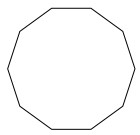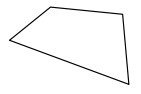# Naming polygons

Polygons are 2-dimensional closed shapes made of straight lines.

In this lesson we learn how to name a polygon by its number of sides. This requires that we know the Greek prefixes for numbers and some rules for their use.

For polygons with 3 through 20 sides, we simply add "gon" to the prefixes at the left. For example a polygon with 5 sides is known by the name pentagon which is got by adding a ‘gon’ to Greek prefix ‘penta’ (meaning five). A polygon with three sides is more commonly called a triangle rather than a trigon and a polygon with four sides is more commonly known as a quadrilateral, rather than a tetragon.

Polygon Names
Sides Names
3 Triangle
5 Pentagon
6 Hexagon
7 Heptagon
8 Octagon
9 Nonagon
10 Decagon

Name the following polygon based on its number of sides.### Solution

Step 1:

Given polygon has ten sides.

Step 2:

So it is a Decagon.

Name the following polygon based on its number of sides.### Solution

Step 1:

Given polygon has four sides.

Step 2:

So it is a tetragon or quadrilateral.